# Machines and Combined Rate

Welcome! You are encouraged to register with the site and login (for free). When you register, you support the site and your question history is saved.

Running at the same constant rate, 6 identical machines can produce a total of 33 widgets per hour. At this rate, how many widgets could 14 such machines produce in 3 hours?

Review: Machines and Combined Rate

Explanation

Rates are best expressed in fractions with units. The rate of the 6 equal machines is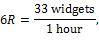where R is the rate of one machine working, since all machines work at an equal rate. To find the rate for 14 machines, not 6, we can multiply both sides of the equation by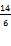: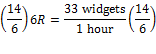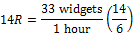We now have 14 machines on the left as we want. The right side is going to give us 6 hours on the right if we multiply it out, but we want 3 hours, so we can multiply the right side by 1 ...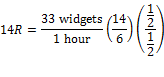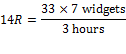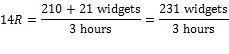For 14 machines, we have 231 widgets in 3 hours.

We can make an estimate to check that this is in the ballpark. The original 6 machines made 33 widgets in 1 hour. And 14 is more than double the number of machines, so they are doing something over 66 widgets an hour. And they are working for 3 hours, so the result will be something like 66 or 70 times 3, so around 210 widgets per hour. Indeed, we could have used that method to start and gotten the answer very quickly, because there is only one answer choice even close to the estimate.